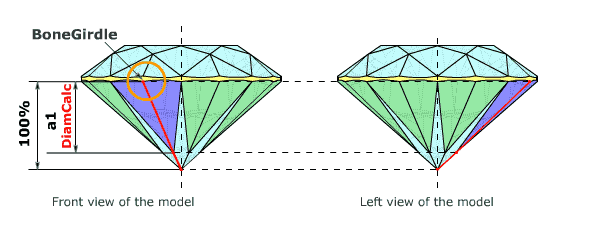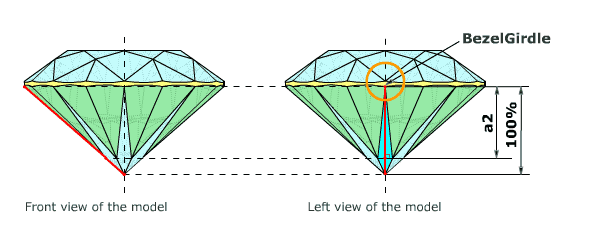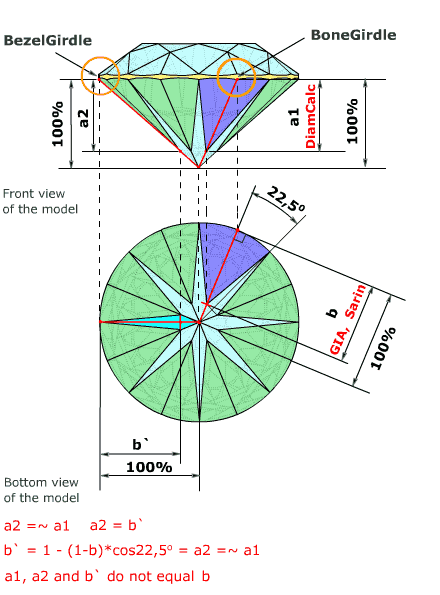Helium help : Measurements are used in different systems and toolsLower girdle facetsApplicable to:

• Brilliant
• Rounded Fancies / Marquise, Oval, Pear

The lower girdle facets are also called Halves. The lower girdle facet length is usually given relative to pavilion main facets length. There are several different ways to measure this parameter giving slightly different results:

1. To measure with vertical projection.
2. To measure with horizontal projection.
3. To measure length differently (non-plane).

To measure with vertical projection

The first way gives the ratio between facets heights. In the strict sense this is not "facet length" but this way is most common and unambiguous (figure 1 and figure 2).

 Figure 1. Measurement of depth girdle facets a1 with vertical projection. Depth girdle facets a1 is facets heights ratio on Bone Girdle. 3DCalc software operate with this option.Figure 2. Measurement of depth girdle facets a2 with vertical projection. Depth girdle facets a2 is facets heights ratio on Bezel Girdle.Depth girdle facets a1 and a2 differ approximately on half difference of thickness girdle in points Bone Girdle and Bezel Girdle (figure 1 and figure 2). It is difference less than 0.1 % for a classical symmetric round brilliant.

To measure with horizontal projection

The second way gives the ratio between facets length, where the lengths are measured in the horizontal projection.

Length girdle facets b is facets length ratio, b` is facets length ratio along the main facet (figure 3).

 Figure 3. Measurement of length girdle facets b and b` with horizontal projection. Length girdle facets is facets length ratio. You may notice, b` is the same number as depth girdle facets a2 (figure 2).For b= 82,0%, b` = 83,4 %, a1~= a2 = 83,4 %
For b = 75,0%, b` = 76,9 %, a1~= a2 = 76,9 %

Depth girdle facets a2 and a1 is more then Length girdle facets b on 1,4 - 1,7(%) approximately.

GIA uses b type of measurements. DiamCalc uses a1 and b types of measurements.

Measurements are used in different systems and tools

1. GIA uses b type of measurements.
2. As far as we know: Sarin uses b type of measurement.
3. OctoNus Helium uses a1 and b types of measurement.
In Helium system a1 is "depth girdle facets", b is "length girdle facets".
4. OctoNus DiamCalc uses a1 and b types of measurement.
In DiamCalc software a1 is "lower facets depth", b is "lower facets length".

Table 1. Names of measurements are used in different systems and tools.

 Type of measurements GIA Sarin Helium (OctoNus) DiamCalc (OctoNus) a1 Depth girdle facets Lower facets depth a2 b Lower girdle length Lower girdle facets Length girdle facets Lower facets lengthTable 2. Values of measurements are used in different systems and tools.

a) b = 75%

 Type of measurements GIA Sarin Helium (OctoNus) DiamCalc (OctoNus) a1 76,9% 76,9% b 75% 75% 75% 75%

b) b = 80%

 Type of measurements GIA Sarin Helium (OctoNus) DiamCalc (OctoNus) a1 81,5% 81,5% b 80% 80% 80% 80%

c) b = 85%

 Type of measurements GIA Sarin Helium (OctoNus) DiamCalc (OctoNus) a1 86,1% 86,1% b 85% 85% 85% 85%